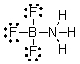# [Solved] What Are the Formal Charges on Boron and Fluorine in the Following

Question 27
Multiple Choice

## What are the formal charges on boron and fluorine in the following structure?A) B = 1+, N = 1+
B) B = 1+, N = 1-
C) B = 1-, N = 1-
D) B = 1-, N = 1+
E) B = 1-, N = 0

10+ million students use Quizplus to study and prepare for their homework, quizzes and exams through 20m+ questions in 300k quizzes.

### Chemistry

Explore our library and get Organic Chemistry Homework Help with various study sets and a huge amount of quizzes and questions

145

Study sets

3.2K

Quizzes

275.8K

Questions

Upload material to get free accessInvite a friend and get free accessSubscribe and get an instant access